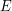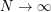## On the link between small ball probabilities and the quantization problem for Gaussian measures on Banach spaces

 Title On the link between small ball probabilities and the quantization problem for Gaussian measures on Banach spaces Publication Type Journal Article Year of Publication 2003 Authors Steffen Dereich, F. Fehringer, A. Matoussi, and M. Scheutzow Journal Journal of Theoretical Probability Volume 16(1) Keywords Gaussian process, High-resolution quantization, isoperimetric inequality., small ball probability Abstract Let m be a centered Gaussian measure on a separable Banach spaceanda positive integer. We study the asymptotics asof the quantization error, i.e., the infimum over all subsetsofof cardinalityof the average distance w.r.t.to the closest point in the set. We compare the quantization error with the average distance which is obtained when the setis chosen by takingi.i.d. copies of random elements with law. Our approach is based on the study of the asymptotics of the measure of a small ball around. Under slight conditions on the regular variation of the small ball function, we get upper and lower bounds of the deterministic and random quantization error and are able to show that both are of the same order. Our conditions are typically satisfied in case the Banach space is infinite dimensional.